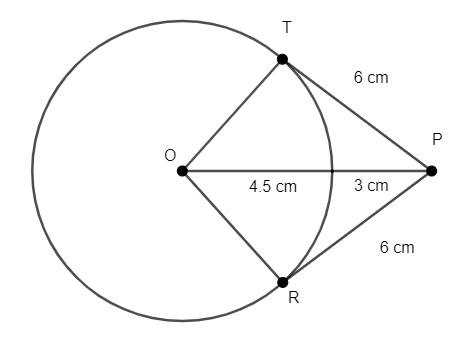Courses
Courses for Kids
Free study material
Free LIVE classes
MoreLIVE
Join Vedantu’s FREE Mastercalss

# Draw a circle of diameter 9 cm. Mark a point at a distance of 7.5 cm from the center of the circle. Draw tangents to the given circle from this exterior point. Measure the length of each tangent.Verified
334.8k+ views
Hint: To solve this first we have to write the steps of construction and then draw the figures with respective dimensions. Draw a circle of radius 4.5 and now draw a line from center and from the end point of line draw tangents and now measure the distance.
Given: Diameter = 9cm
Radius = $\dfrac{9}{2}=4.5cm$

Mark a point O. Draw a circle of radius 4.5cm.
From the point mark a point P such OP = 7.5cm
Draw tangents PT and PR from the point P such that it touches the circumference of the circle.From the diagram we can see that a right angled triangle is formed by OTP.
Now by Pythagoras theorem we can find the distance from point P to R which is a tangent to a circle.
${{c}^{2}}={{a}^{2}}+{{b}^{2}}$ . . . . . . . . . . . . . . . . . (a)
${{4.5}^{2}}+{{t}^{2}}={{7.5}^{2}}$
${{t}^{2}}={{7.5}^{2}}-{{4.5}^{2}}$
${{t}^{2}}=56.25-20.25$
${{t}^{2}}=36$
$t=6$
t is the distance from P to R.
Length of the tangents PT = 6cm and PR = 6 cm.

Note: The right angle is at vertex T. There are two tangents drawn from a point and they have the same distance if they are projected on a circle. The tangents are the lines which just touch the circle. All dimensions are in cm.
Last updated date: 27th Sep 2023
Total views: 334.8k
Views today: 4.34k